# 2nd Order Low-Pass Filter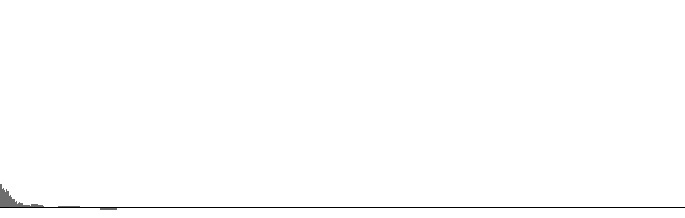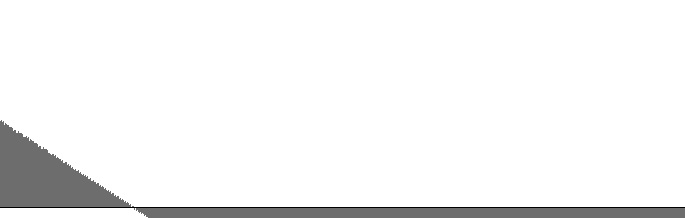Figure 1 (a): FilterBandPass2 amplitude versus log(frequency) with 0 Hz peak frequency and 6 Hz. bandwidth. Figure 1 (b): FilterBandPass2 log(amplitude) versus log(frequency) with 0 Hz peak frequency and 6 Hz. bandwidth.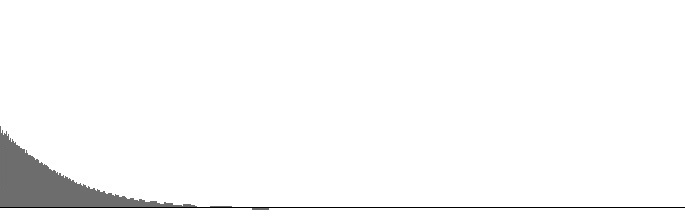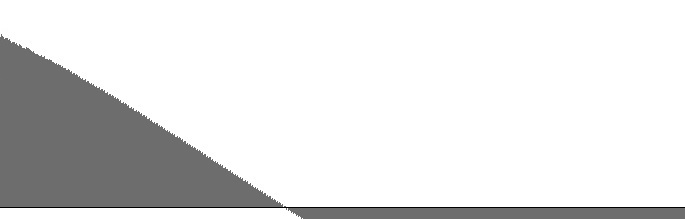Figure 2 (a): FilterBandPass2 amplitude versus log(frequency) with 0 Hz peak frequency and 25 Hz. bandwidth. Figure 2 (b): FilterBandPass2 log(amplitude) versus log(frequency) with 0 Hz peak frequency and 25 Hz. bandwidth.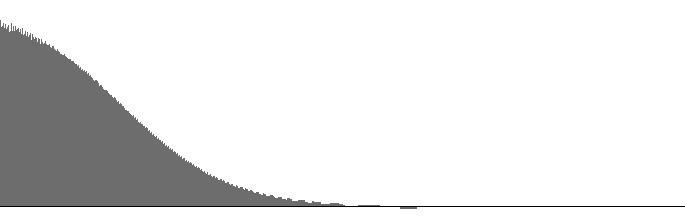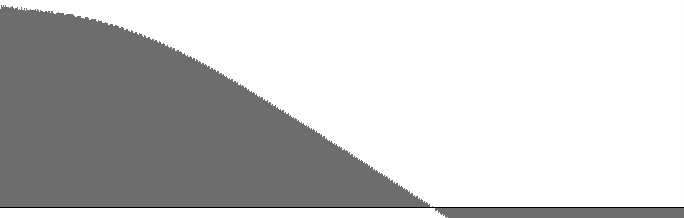Figure 3 (a): FilterBandPass2 amplitude versus log(frequency) with 0 Hz peak frequency and 100 Hz. bandwidth. Figure 3 (b): FilterBandPass2 log(amplitude) versus log(frequency) with 0 Hz peak frequency and 100 Hz. bandwidth.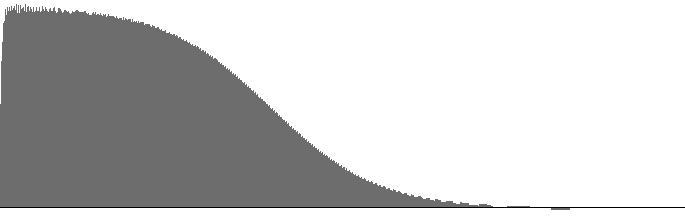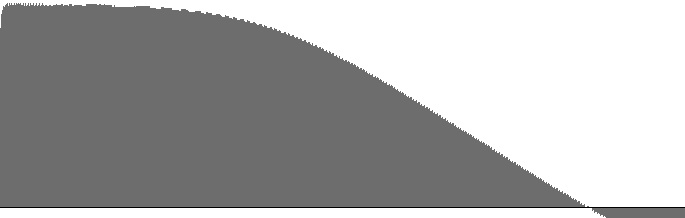Figure 4 (a): FilterBandPass2 amplitude versus log(frequency) with 0 Hz peak frequency and 400 Hz. bandwidth. Figure 4 (b): FilterBandPass2 log(amplitude) versus log(frequency) with 0 Hz peak frequency and 400 Hz. bandwidth.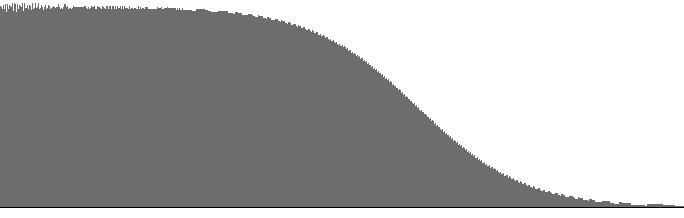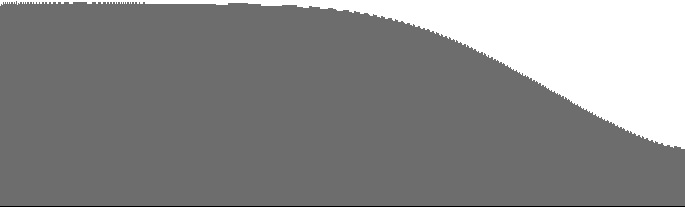Figure 5 (a): FilterBandPass2 amplitude versus log(frequency) with 0 Hz peak frequency and 1600 Hz. bandwidth. Figure 5 (b): FilterBandPass2 log(amplitude) versus log(frequency) with 0 Hz peak frequency and 1600 Hz. bandwidth.

The FilterBandPass2 unit actually implements a 2nd order resonance filter, but the unit can be employed in low-pass mode if the peak frequency is set to zero. In this circumstance the bandwidth input determines the cutoff frequency. Figures 1 (a) through 5 (b) plot frequency responses for the FilterBandPass2 unit in low-pass mode.

The dropoff rate in decibels per octave is discernable as the slope in the (b) graphs. The steepest dropoff rate is around -3.1 dB per octave; however, notice that this slope appears in all (b) graphs.

One might expect the slope of the (b) graphs to decrease as the cutoff frequency increases, but this is not the case. Rather, there is a region of flat response which first becomes apparent above 25 Hz. and which expands rightward as the cutoff frequency increases. This is in fact the behavior normally expected of a low-pass filter, but for the specific purpose of generating colored noise, the behavior is inconvenient.

At 25 Hz. bandwidth, the dropoff rate is slightly steeper than that of “pink” or “(1/f)” noise as described in Wikipedia.

For bandwidths narrower than 25 Hz., differences between frequency-response curves are mostly a matter of gain. Bandwidths narrower than 25 Hz. are therefore not recommended.

 © Charles Ames Page created: 2014-02-20 Last updated: 2015-07-12